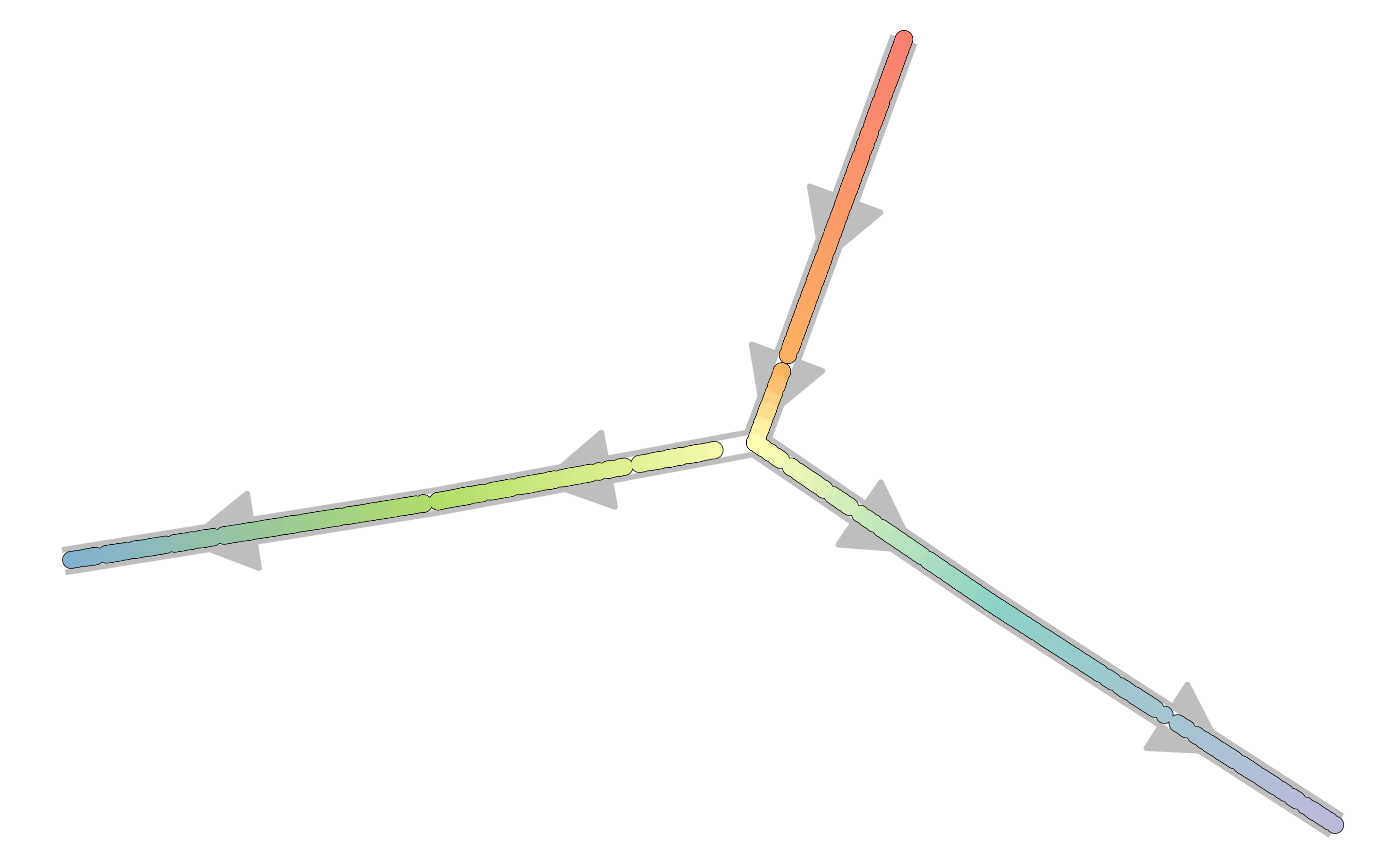This vignette demonstrates the basics of running a dyngen simulation. If you haven’t done so already, first check out the installation instructions in the README.

### Step 1: Define backbone and other parameters

A dyngen simulation can be started by providing a backbone to the initialise_model() function. The backbone of a dyngen model is what determines the overall dynamic process that a cell will undergo during a simulation. It consists of a set of gene modules, which regulate eachother in such a way that expression of certain genes change over time in a specific manner.

library(tidyverse)
library(dyngen)

set.seed(1)

backbone <- backbone_bifurcating()
config <-
initialise_model(
backbone = backbone,
num_tfs = nrow(backbone$module_info), num_targets = 500, num_hks = 500, verbose = FALSE ) # the simulation is being sped up because rendering all vignettes with one core # for pkgdown can otherwise take a very long time set.seed(1) config <- initialise_model( backbone = backbone, num_cells = 1000, num_tfs = nrow(backbone$module_info),
num_targets = 50,
num_hks = 50,
verbose = FALSE,
simulation_params = simulation_default(
total_time = 1000,
census_interval = 2,
ssa_algorithm = ssa_etl(tau = 300/3600),
experiment_params = simulation_type_wild_type(num_simulations = 10)
)
)
plot_backbone_statenet(config)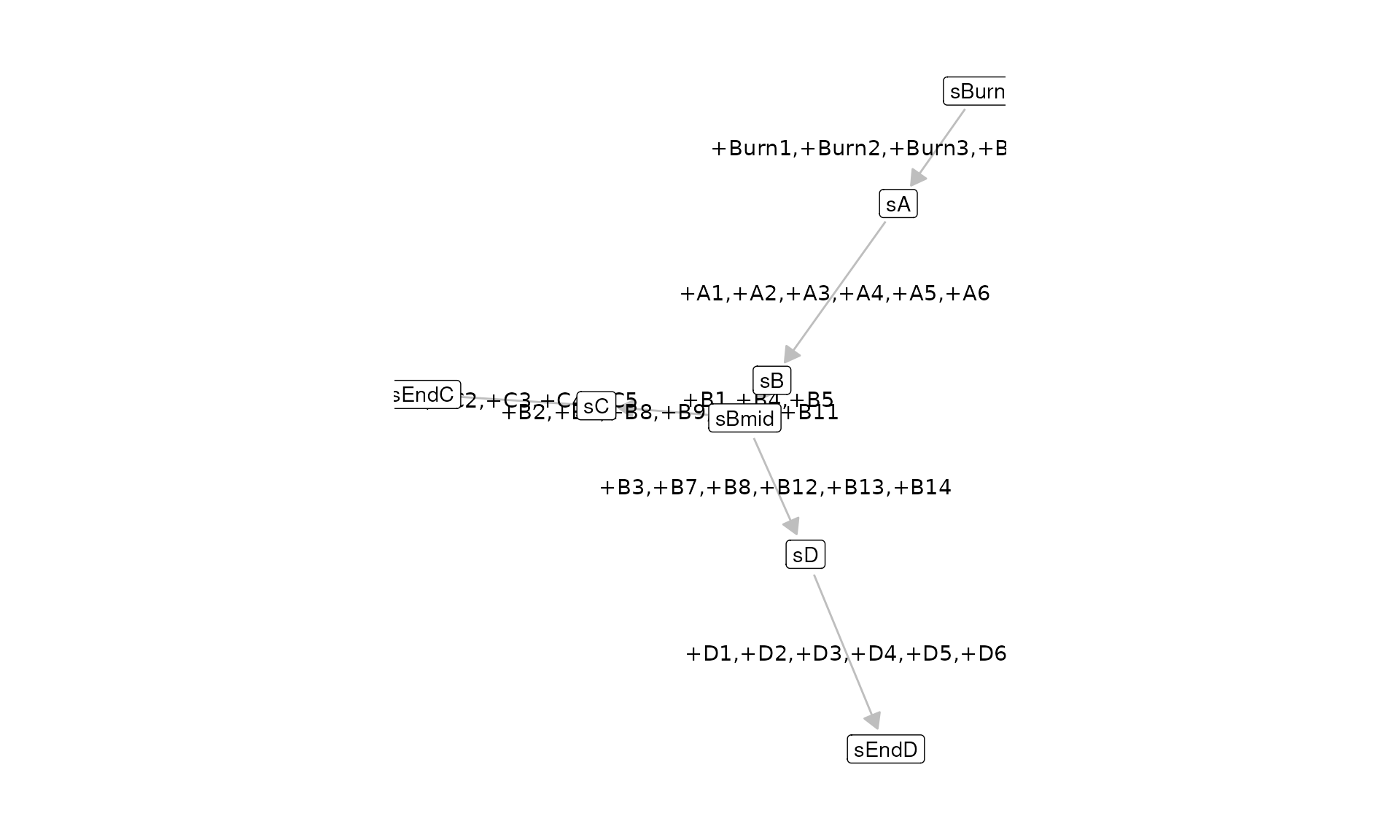plot_backbone_modulenet(config)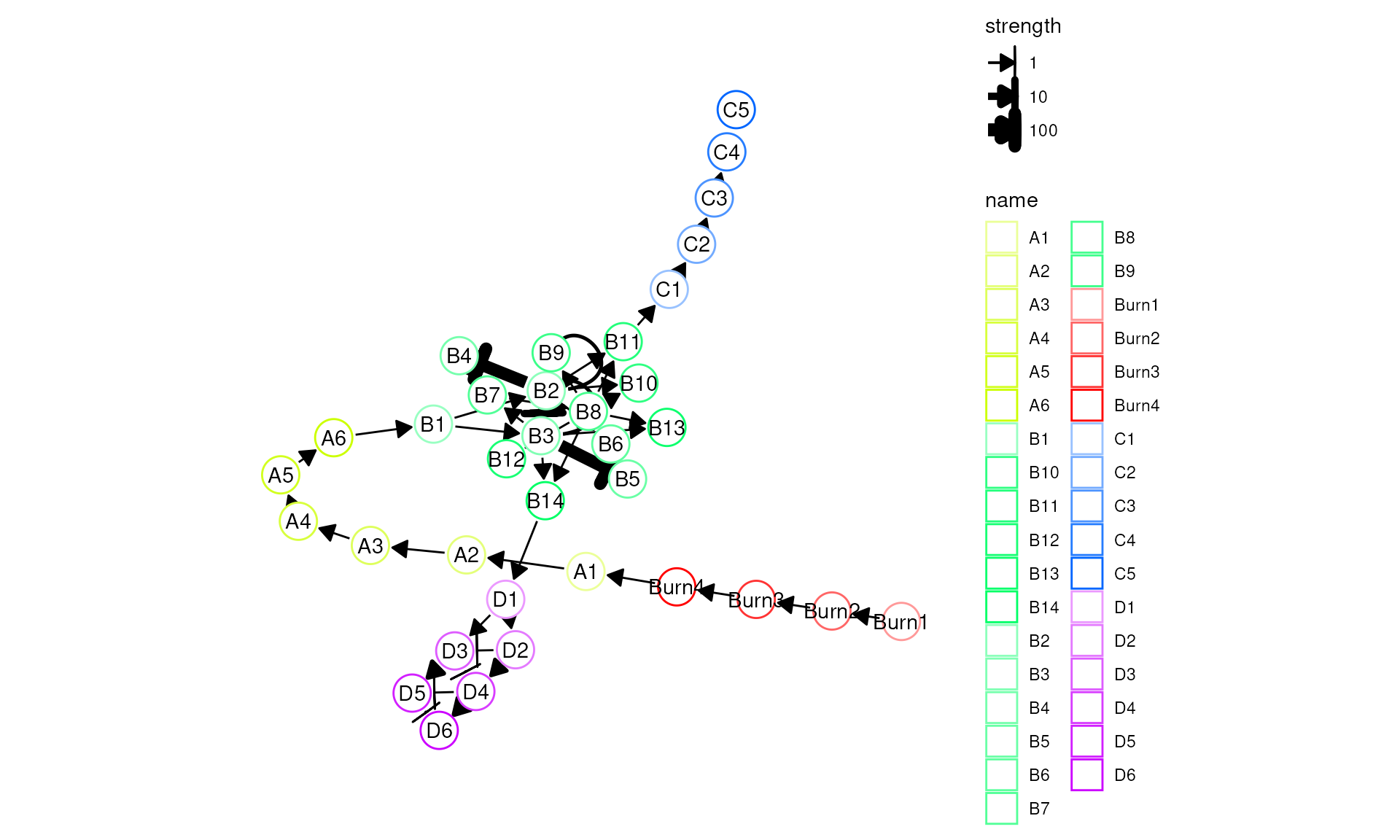For backbones with all different sorts of topologies, check list_backbones():

names(list_backbones())
##   "bifurcating"             "bifurcating_converging"
##   "bifurcating_cycle"       "bifurcating_loop"
##   "binary_tree"             "branching"
##   "consecutive_bifurcating" "converging"
##   "cycle"                   "cycle_simple"
##  "disconnected"            "linear"
##  "linear_simple"           "trifurcating"

### Step 2: Generate transcription factors (TFs)

Each gene module consists of a set of transcription factors. These can be generated and visualised as follows.

model <- generate_tf_network(config)
plot_feature_network(model, show_targets = FALSE)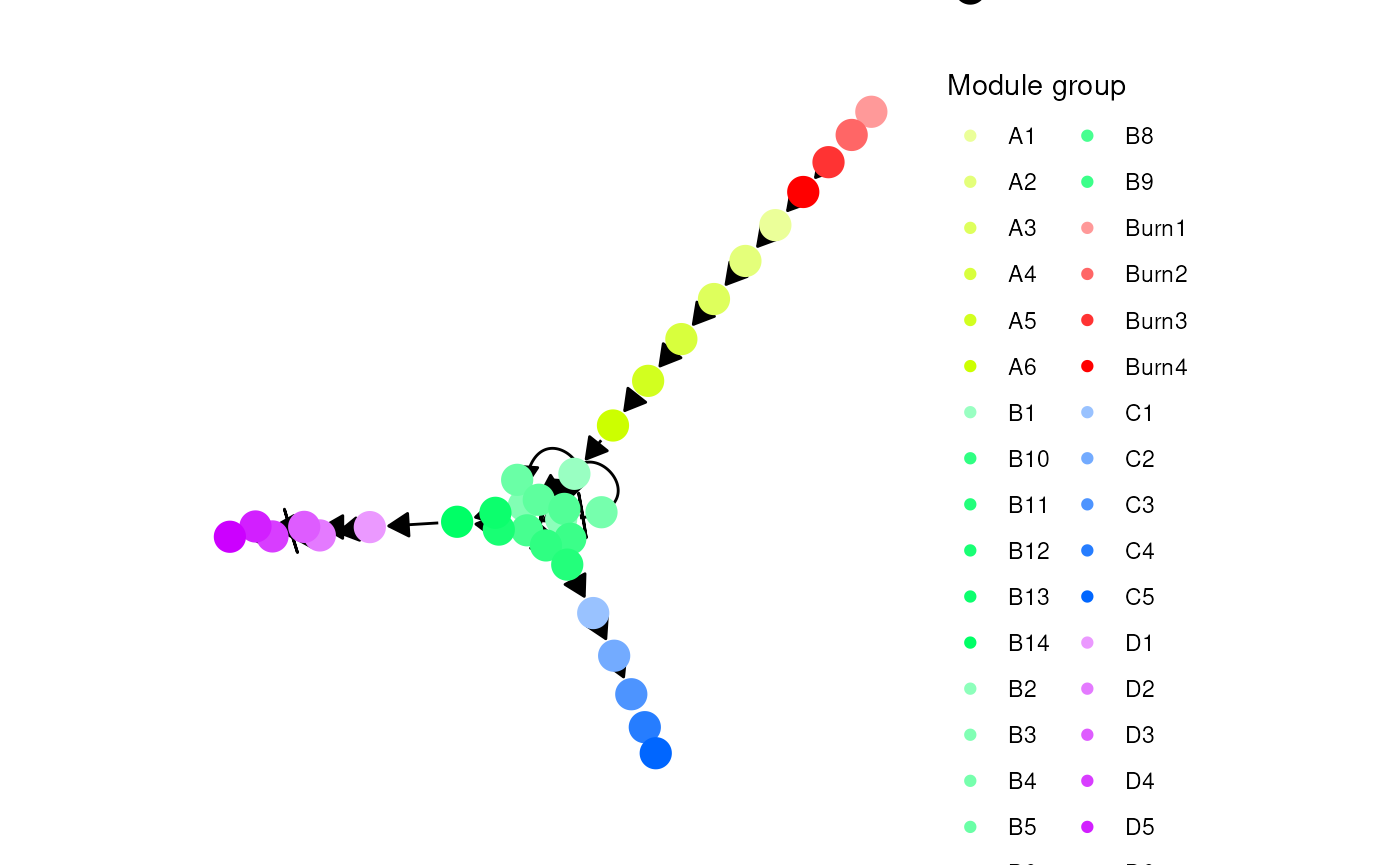### Step 3: Sample target genes and housekeeping genes (HKs)

Next, target genes and housekeeping genes are added to the network by sampling a gold standard gene regulatory network using the Page Rank algorithm. Target genes are regulated by TFs or other target genes, while HKs are only regulated by themselves.

model <- generate_feature_network(model)
plot_feature_network(model)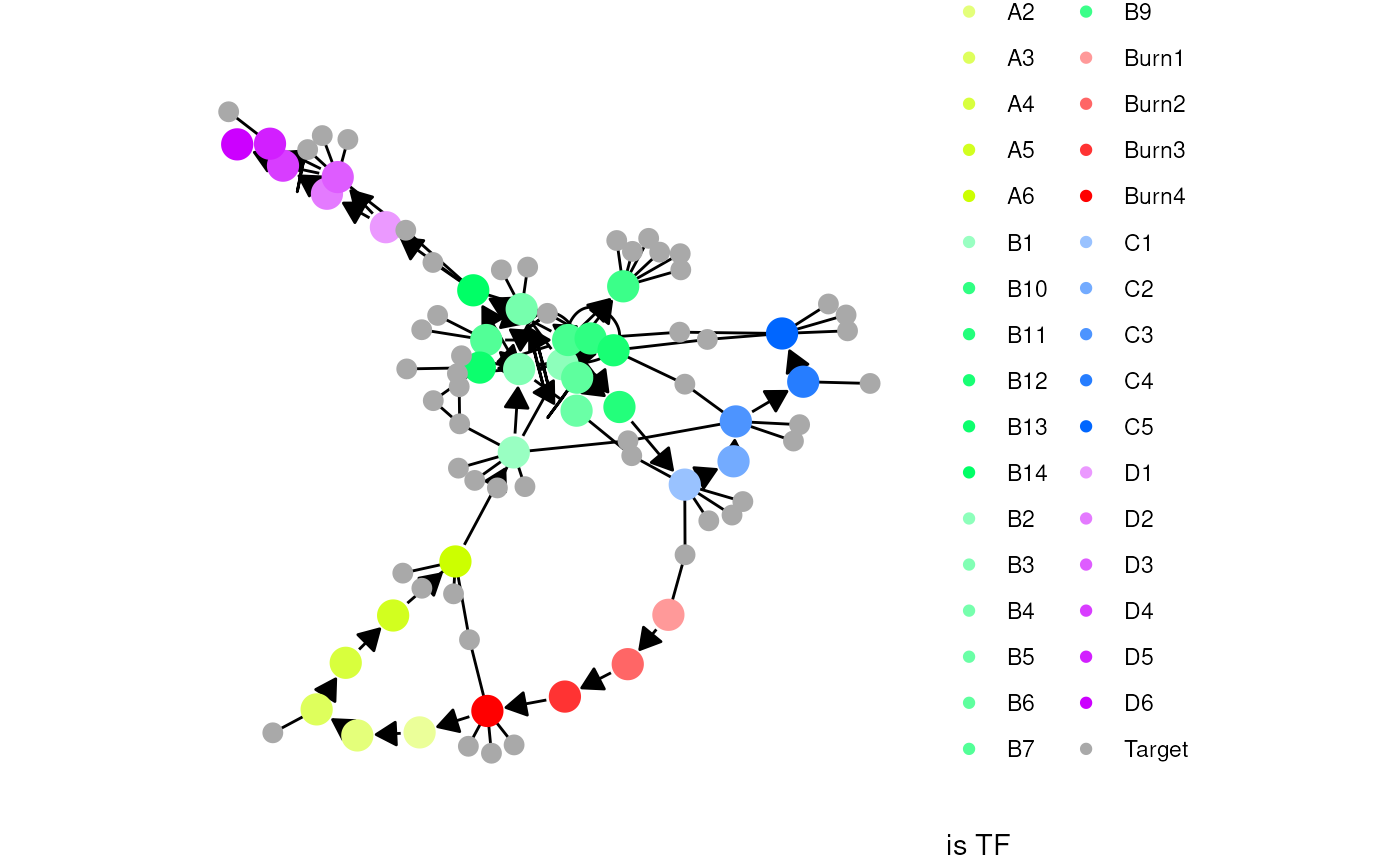plot_feature_network(model, show_hks = TRUE)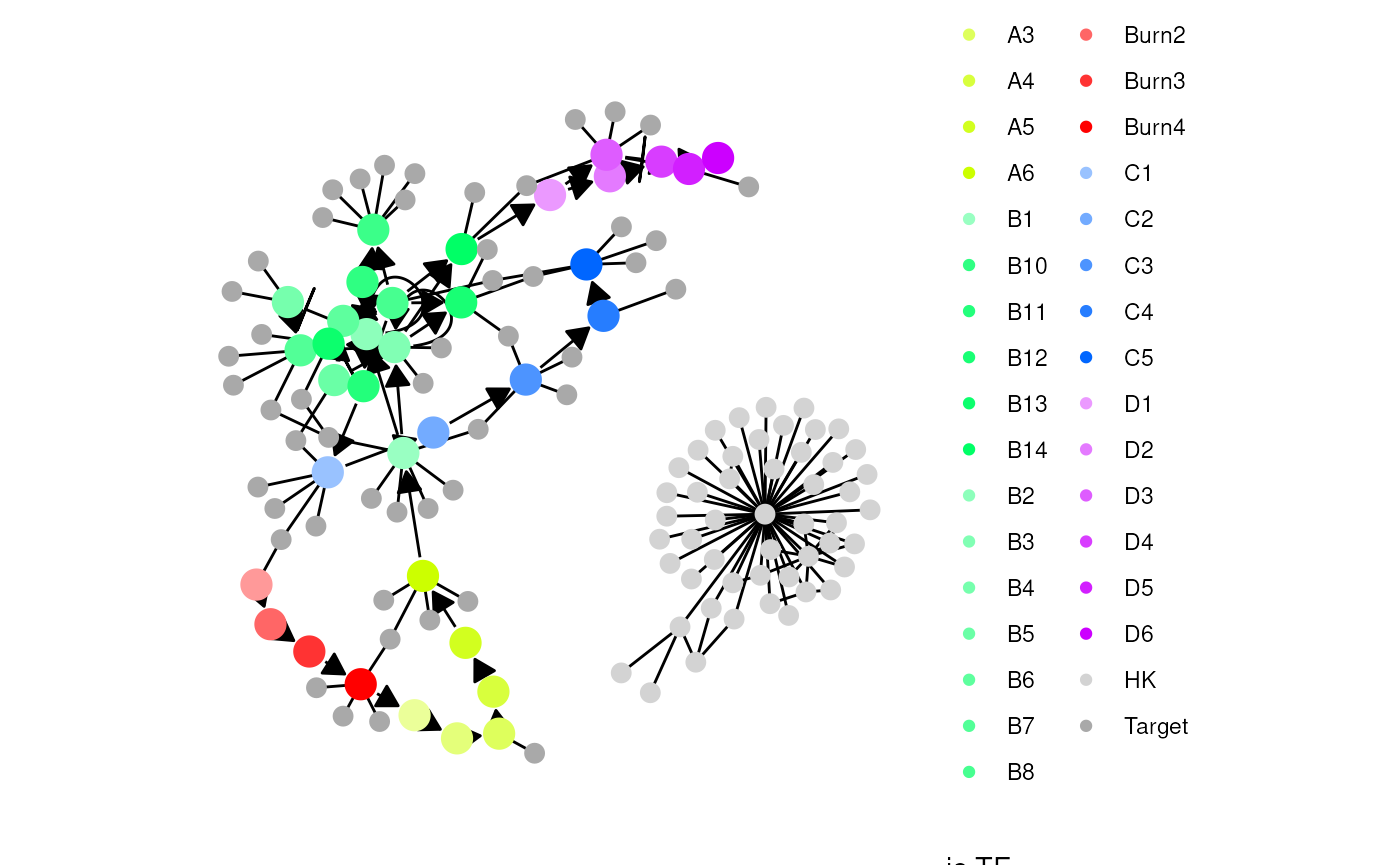### Step 4: Generate kinetics

Note that the target network does not show the effect of some interactions, because these are generated along with other kinetics parameters of the SSA simulation.

model <- generate_kinetics(model)
plot_feature_network(model)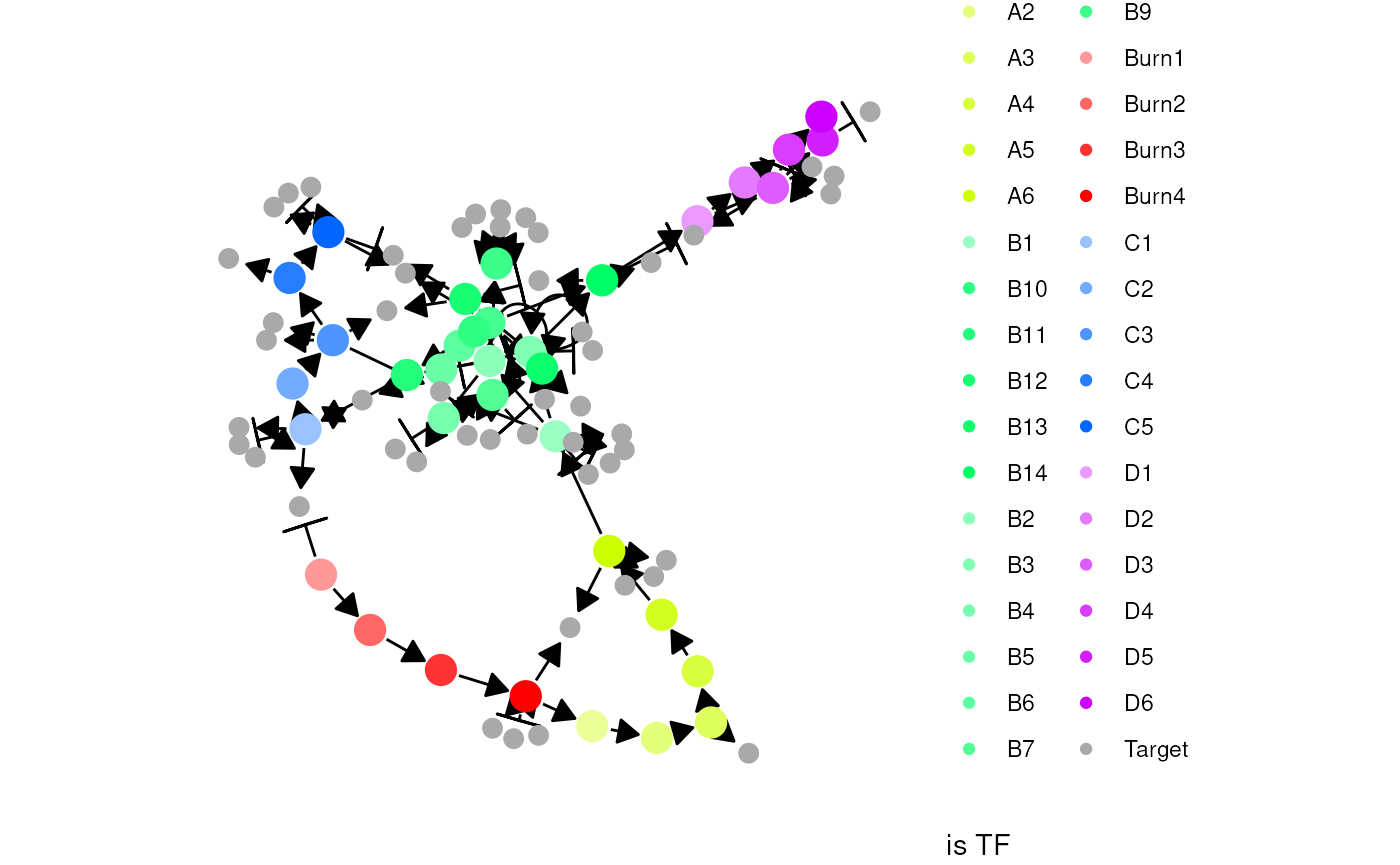plot_feature_network(model, show_hks = TRUE)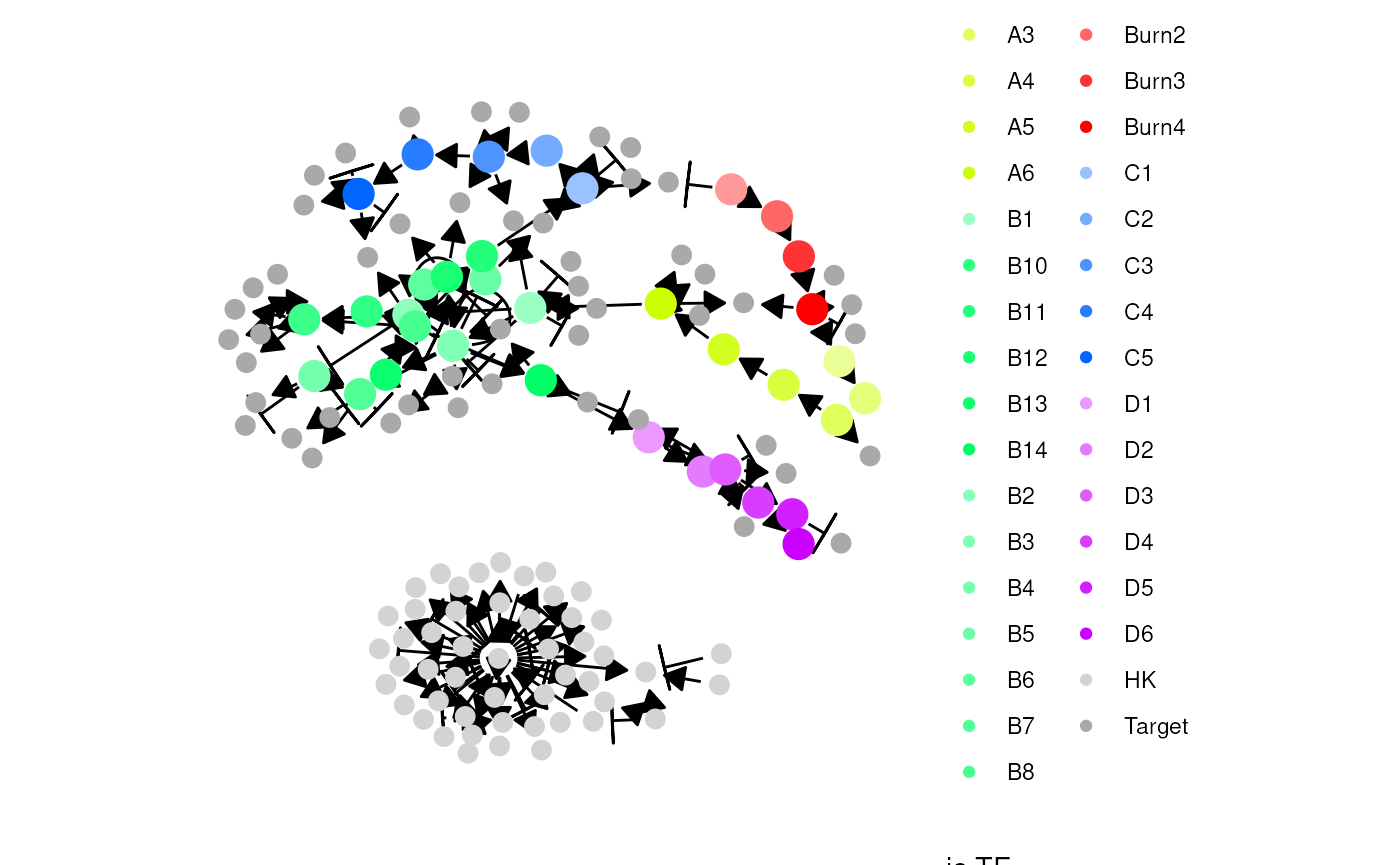### Step 5: Simulate gold standard

The gold standard is simulated by enabling certain parts of the module network and performing ODE simulations. The gold standard are visualised by performing a dimensionality reduction on the mRNA expression values.

model <- generate_gold_standard(model)
plot_gold_simulations(model) + scale_colour_brewer(palette = "Dark2")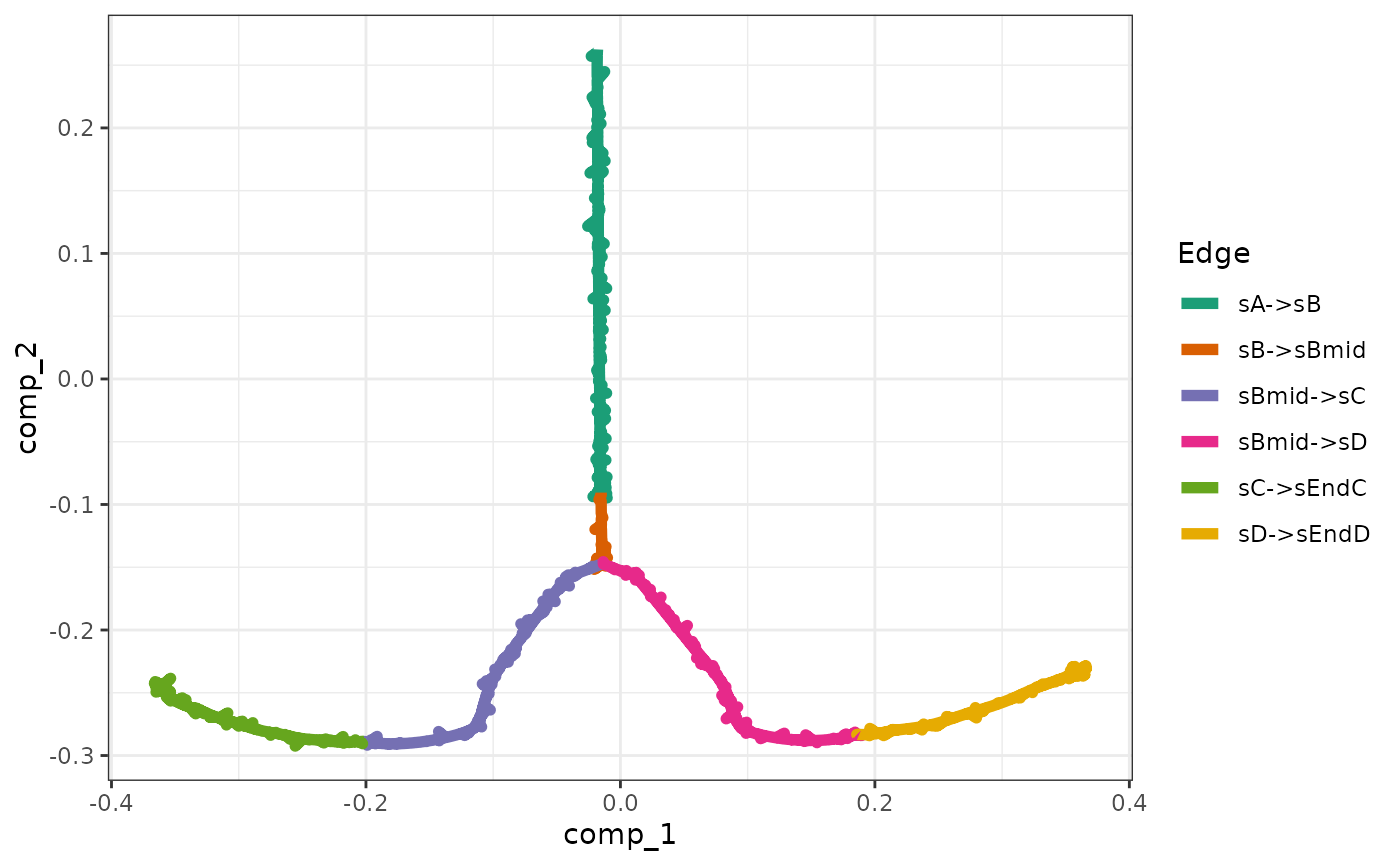The expression of the modules (average of TFs) can be visualised as follows.

plot_gold_expression(model, what = "mol_mrna") # mrna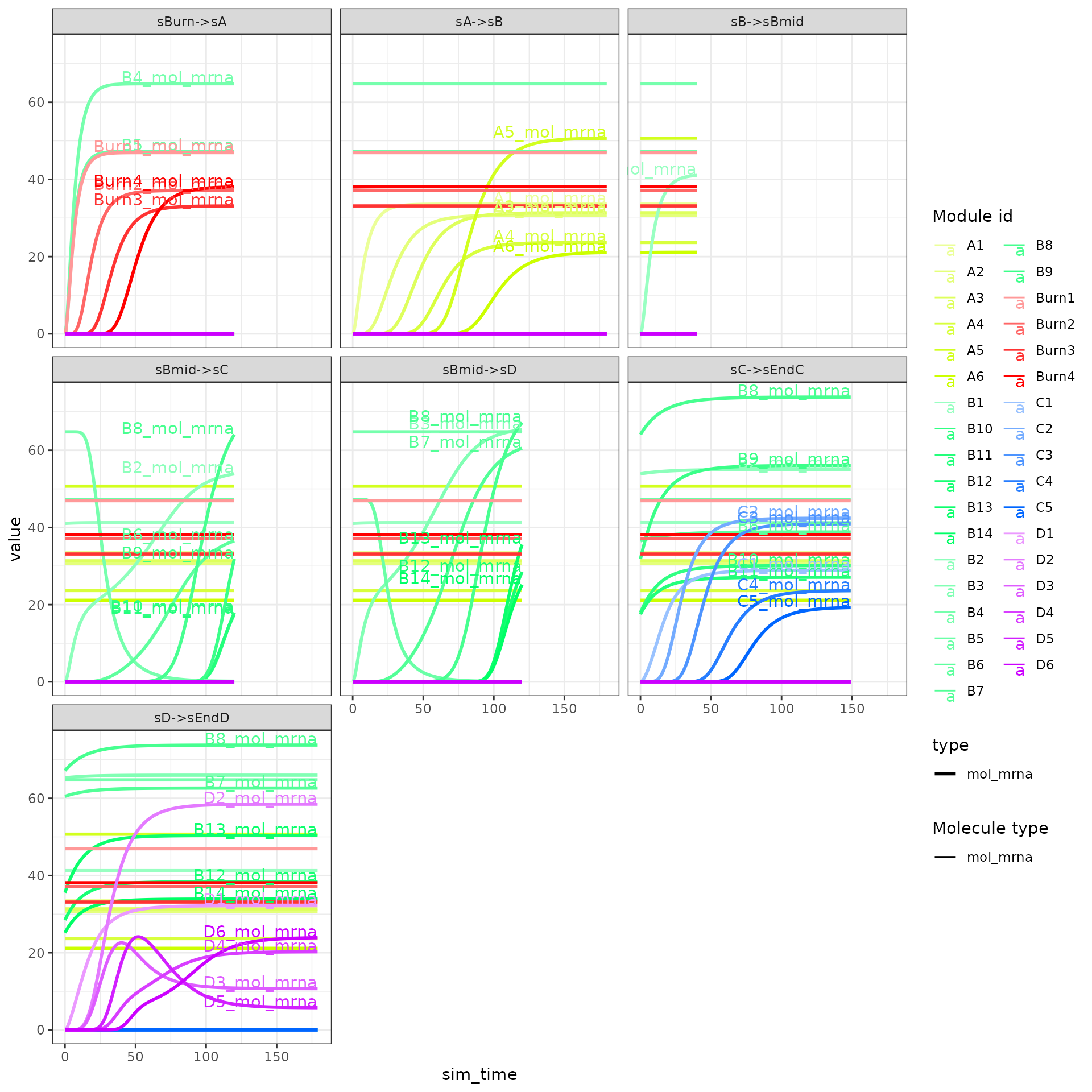plot_gold_expression(model, label_changing = FALSE) # premrna, mrna, and protein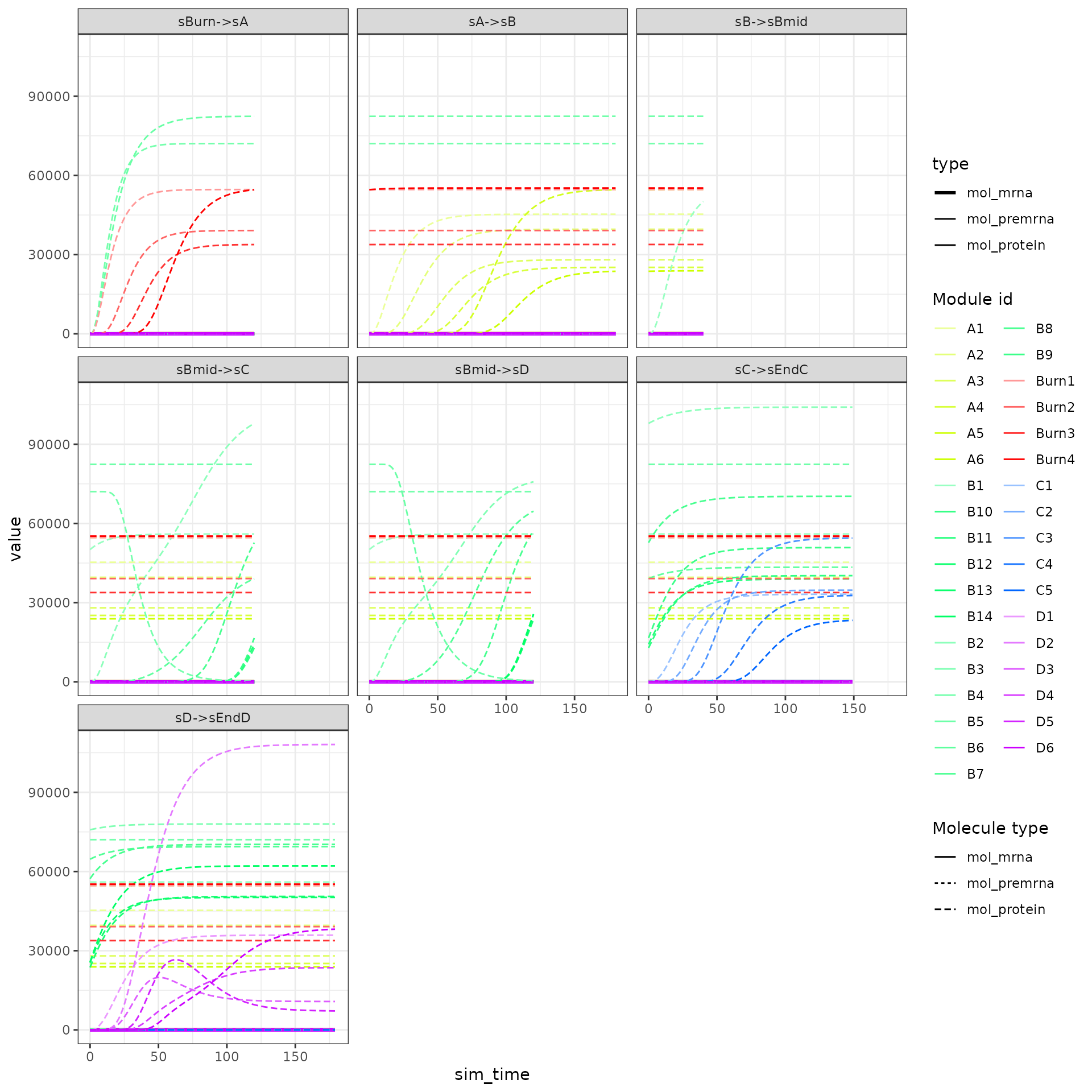### Step 6: Simulate cells.

Cells are simulated by running SSA simulations. The simulations are again using dimensionality reduction.

model <- generate_cells(model)
plot_simulations(model)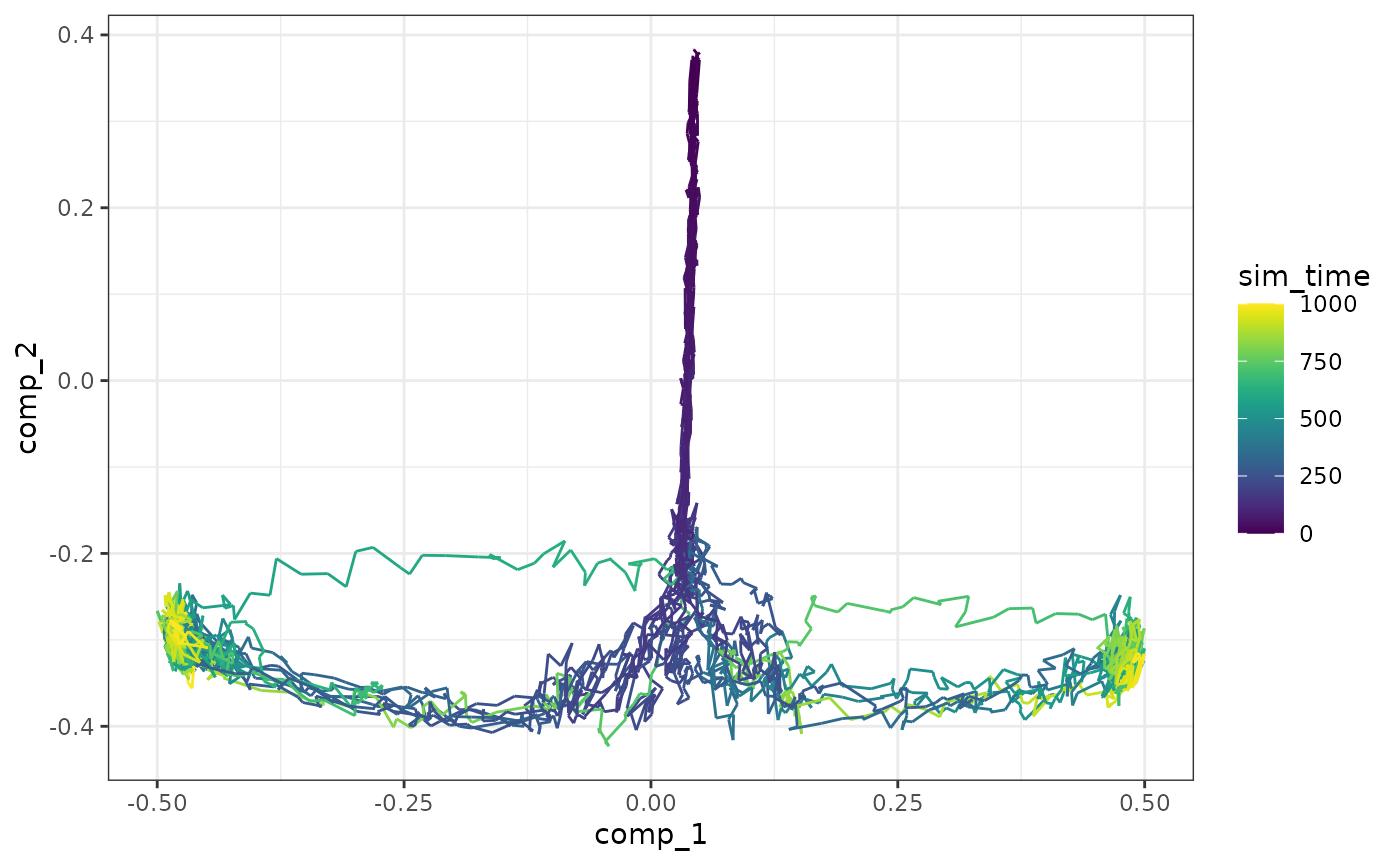The gold standard can be overlayed on top of the simulations.

plot_gold_simulations(model) + scale_colour_brewer(palette = "Dark2")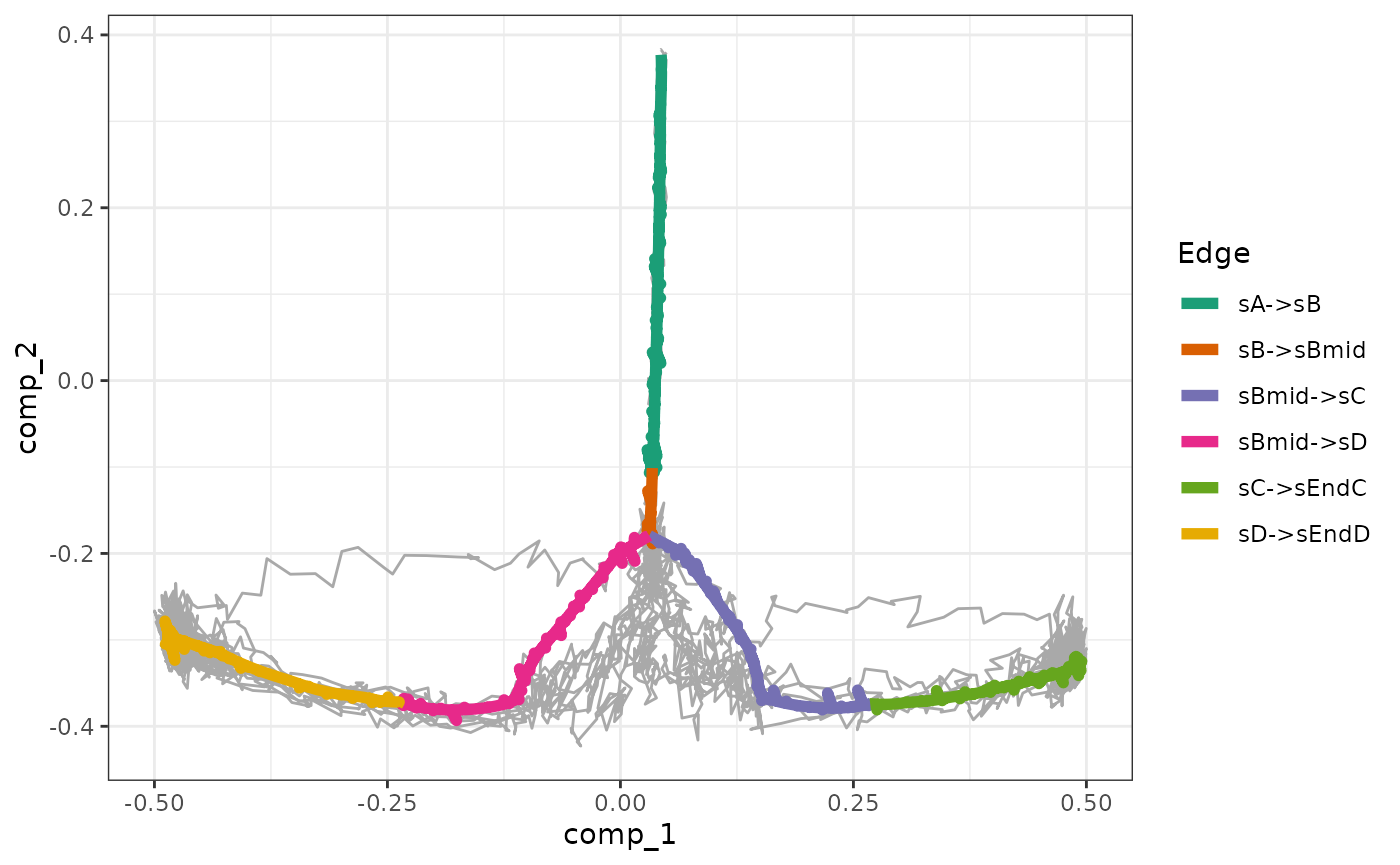We can check how each segment of a simulation is mapped to the gold standard.

plot_gold_mappings(model, do_facet = FALSE) + scale_colour_brewer(palette = "Dark2")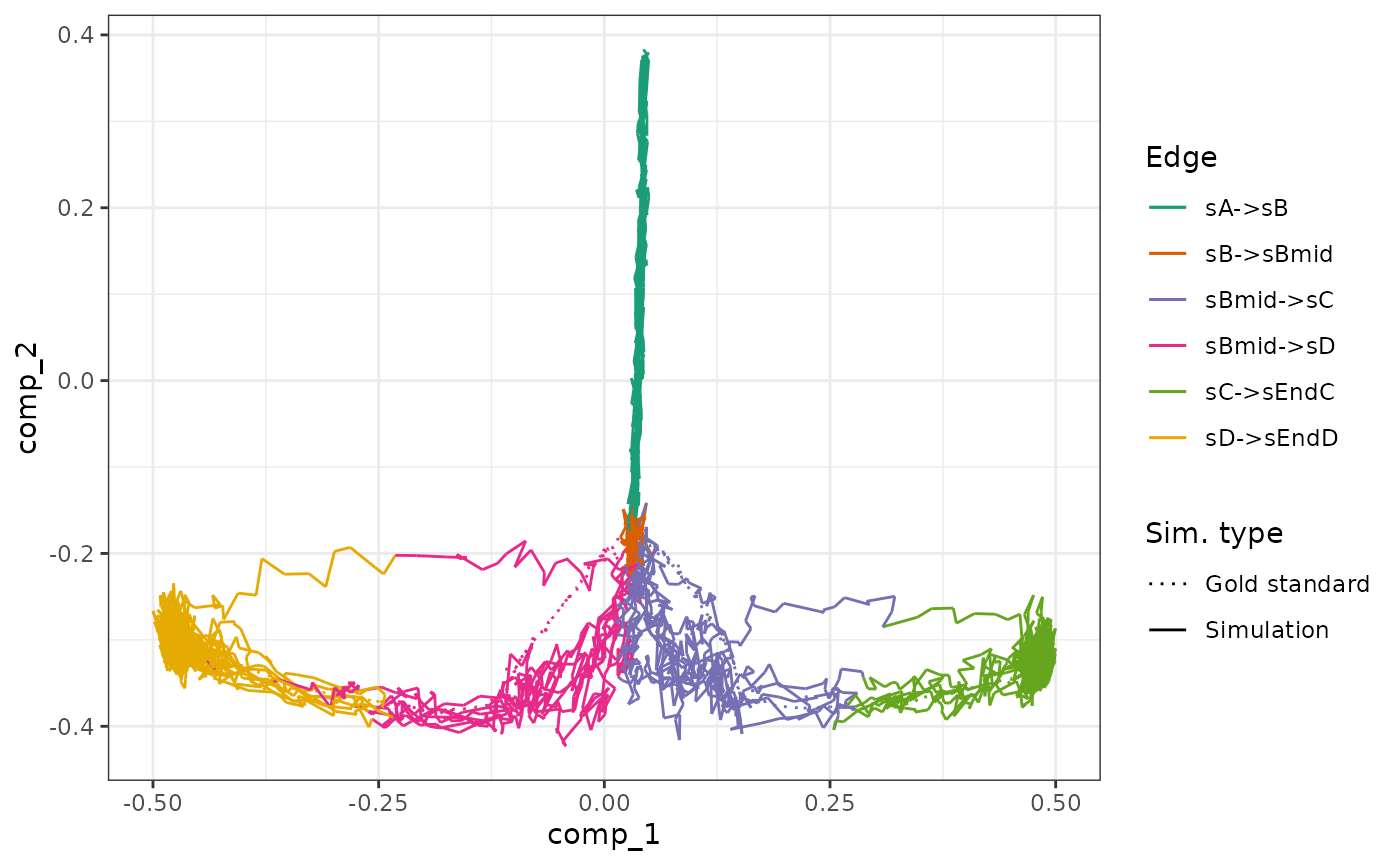The expression of the modules (average of TFs) of a single simulation can be visualised as follows.

plot_simulation_expression(model, 1:4, what = "mol_mrna")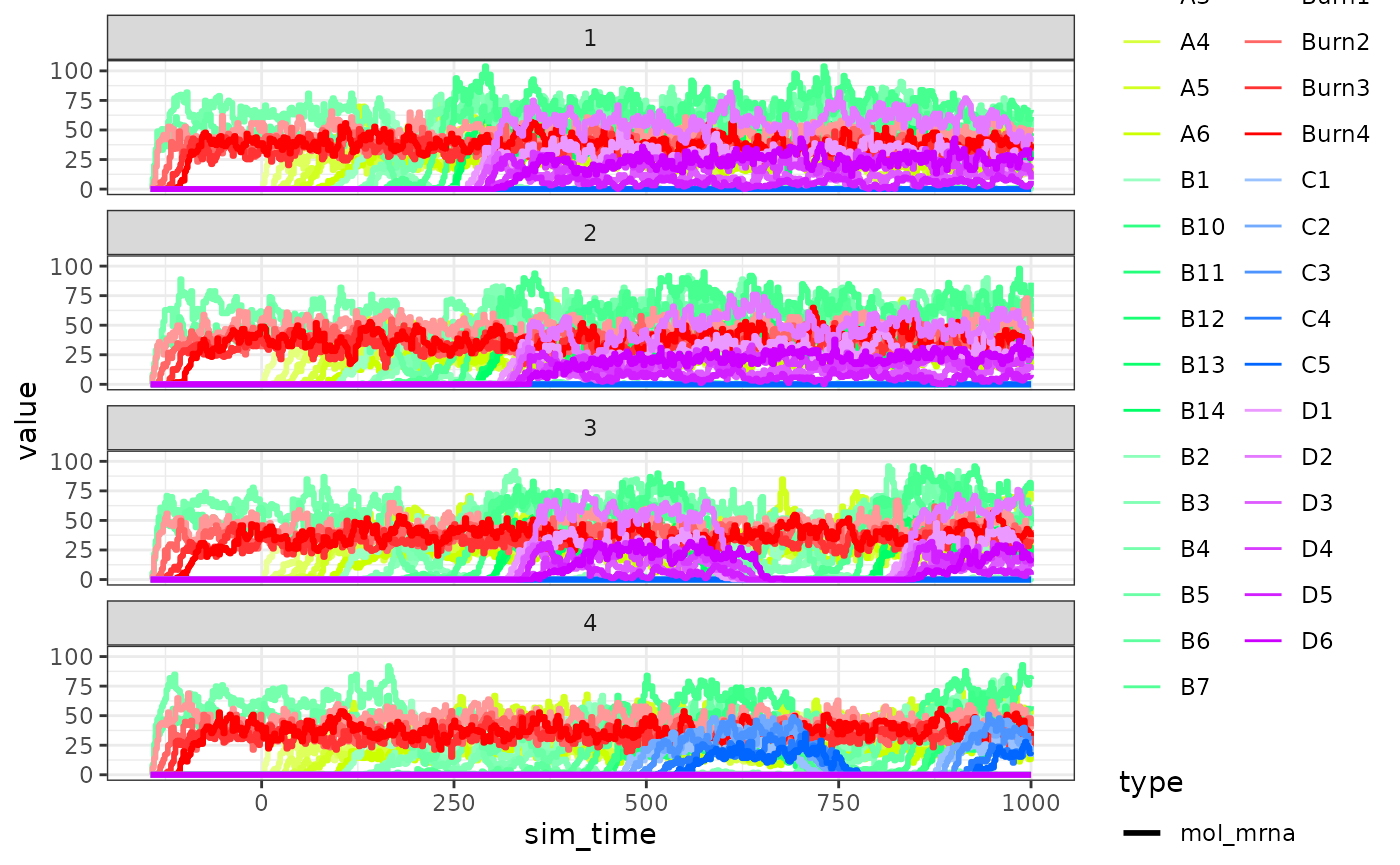### Step 7: Experiment emulation

Effects from performing a single-cell RNA-seq experiment can be emulated as follows.

model <- generate_experiment(model)

### Step 8: Convert to a dyno object

Converting the dyngen to a dyno object allows you to visualise the dataset using the dynplot functions, or infer trajectories using dynmethods.

dataset <- as_dyno(model)
## Loading required namespace: dynwrap

#### Visualise with dynplot

library(dynplot)
plot_dimred(dataset)
## Coloring by milestone
## Using milestone_percentages from trajectory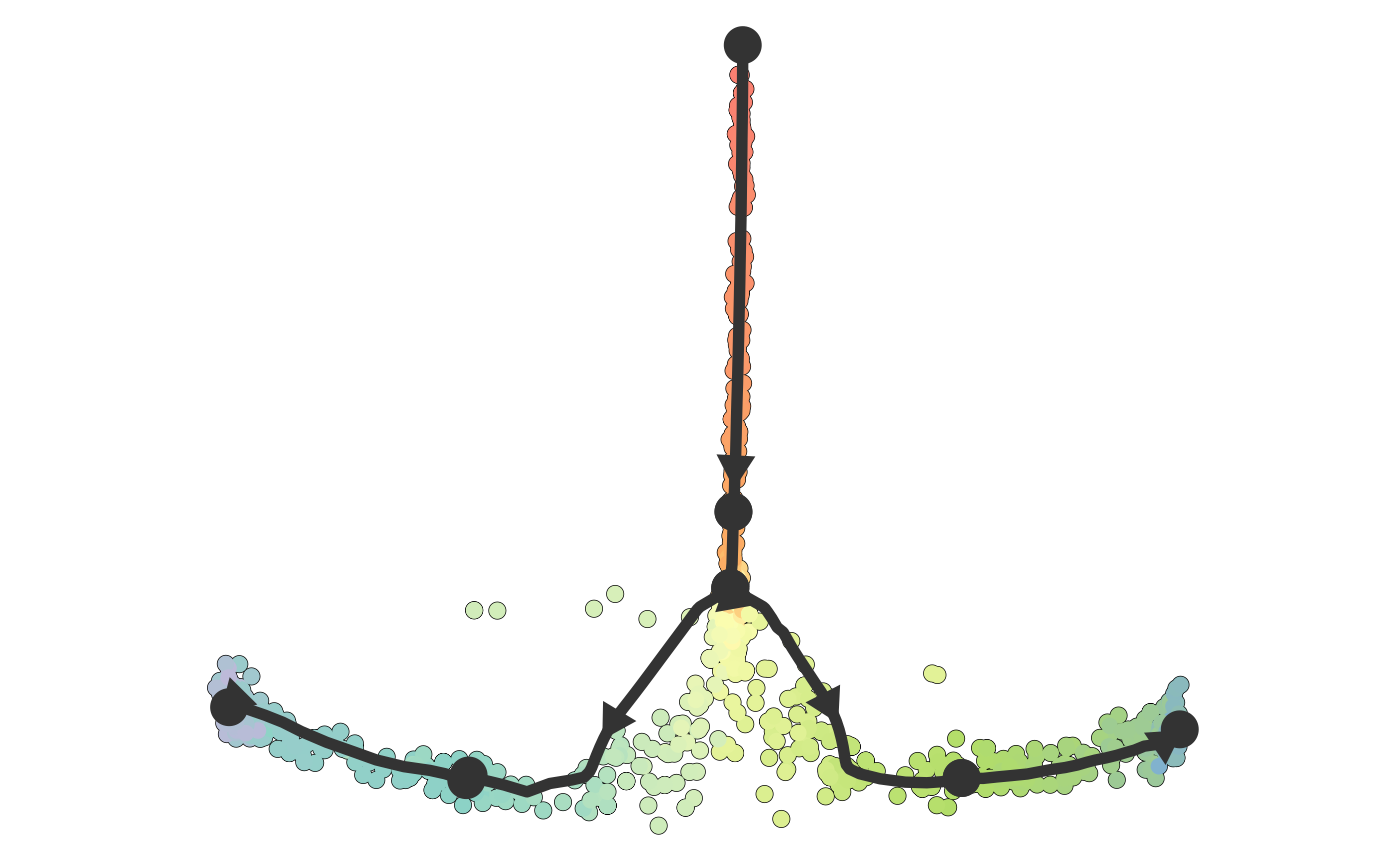plot_graph(dataset)
## Coloring by milestone
## Using milestone_percentages from trajectory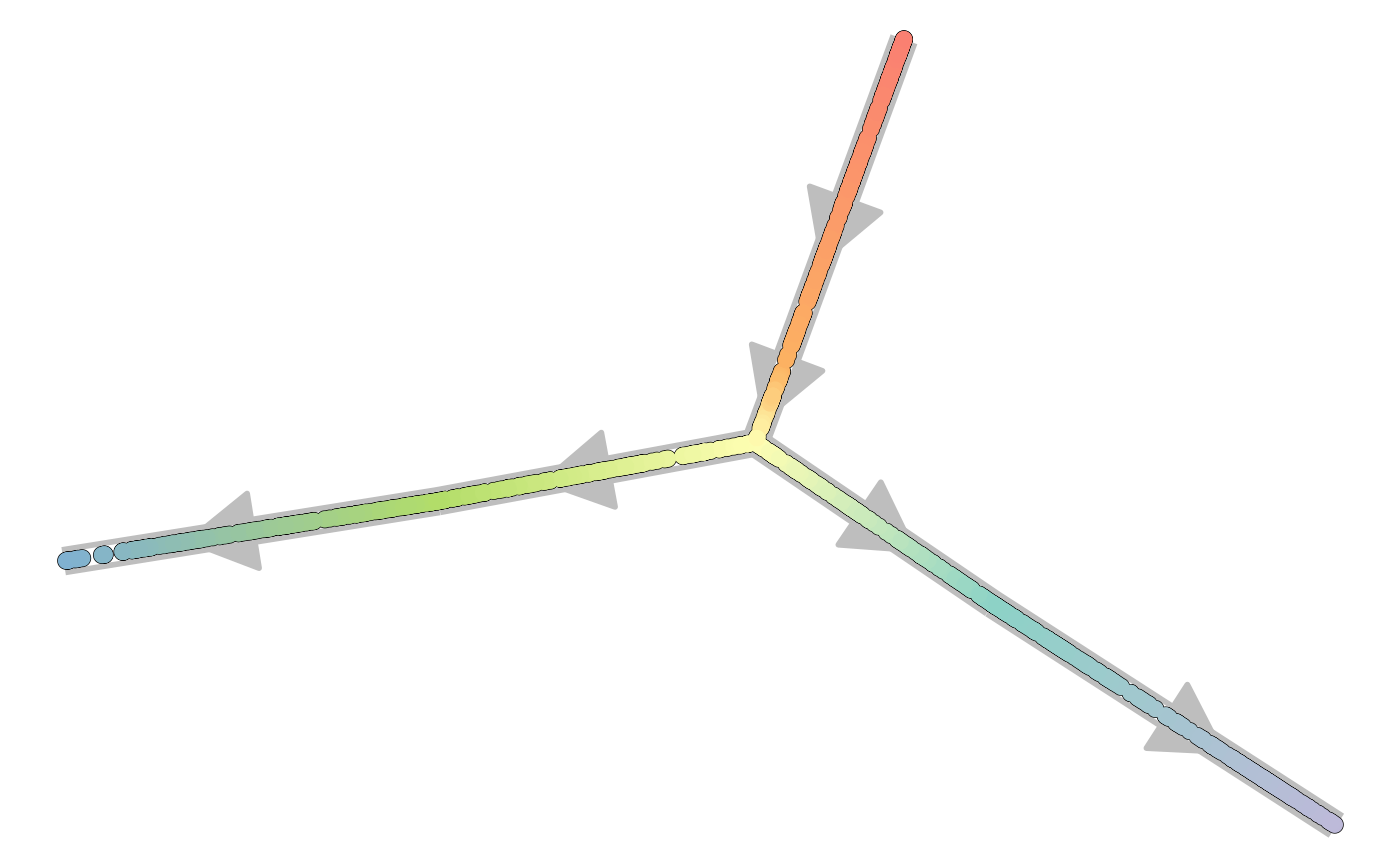#### Save output to file

You can save the output of the model and/or dataset to a file as follows.

write_rds(model, "model.rds", compress = "gz")
write_rds(dataset, "dataset.rds", compress = "gz")

### Step 8 alternative: Convert to an anndata/SCE/Seurat object

dyngen 1.0.0 allows converting the output to an anndata, SCE or Seurat object as well. Check out the anndata documentation on how to install anndata for R.

library(anndata)
ad$write_h5ad("dataset.h5ad") library(SingleCellExperiment) sce <- as_sce(model) write_rds(sce, "dataset_sce.rds") library(Seurat) seurat <- as_seurat(model) write_rds(seurat, "dataset_seurat.rds") ## One-shot function dyngen also provides a one-shot function for running all of the steps all at once and producing plots. out <- generate_dataset( config, format = "dyno", make_plots = TRUE ) dataset <- out$dataset
model <- out$model print(out$plot)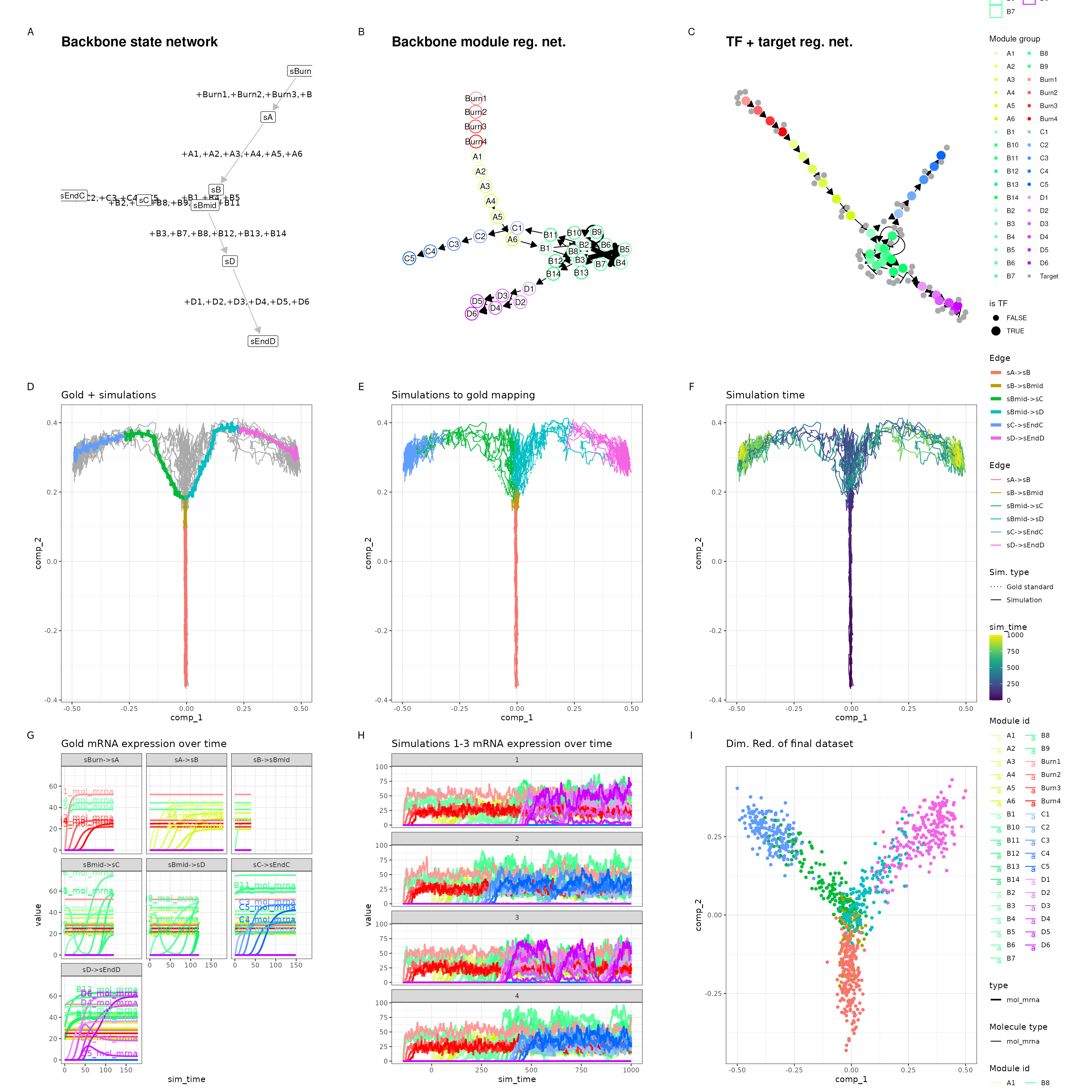dataset and model can be used in much the same way as before.

library(dyno)
## Loading required package: dynfeature
## Loading required package: dynguidelines
## Loading required package: dynmethods
## Loading required package: dynwrap
plot_dimred(dataset)
## Coloring by milestone
## Using milestone_percentages from trajectory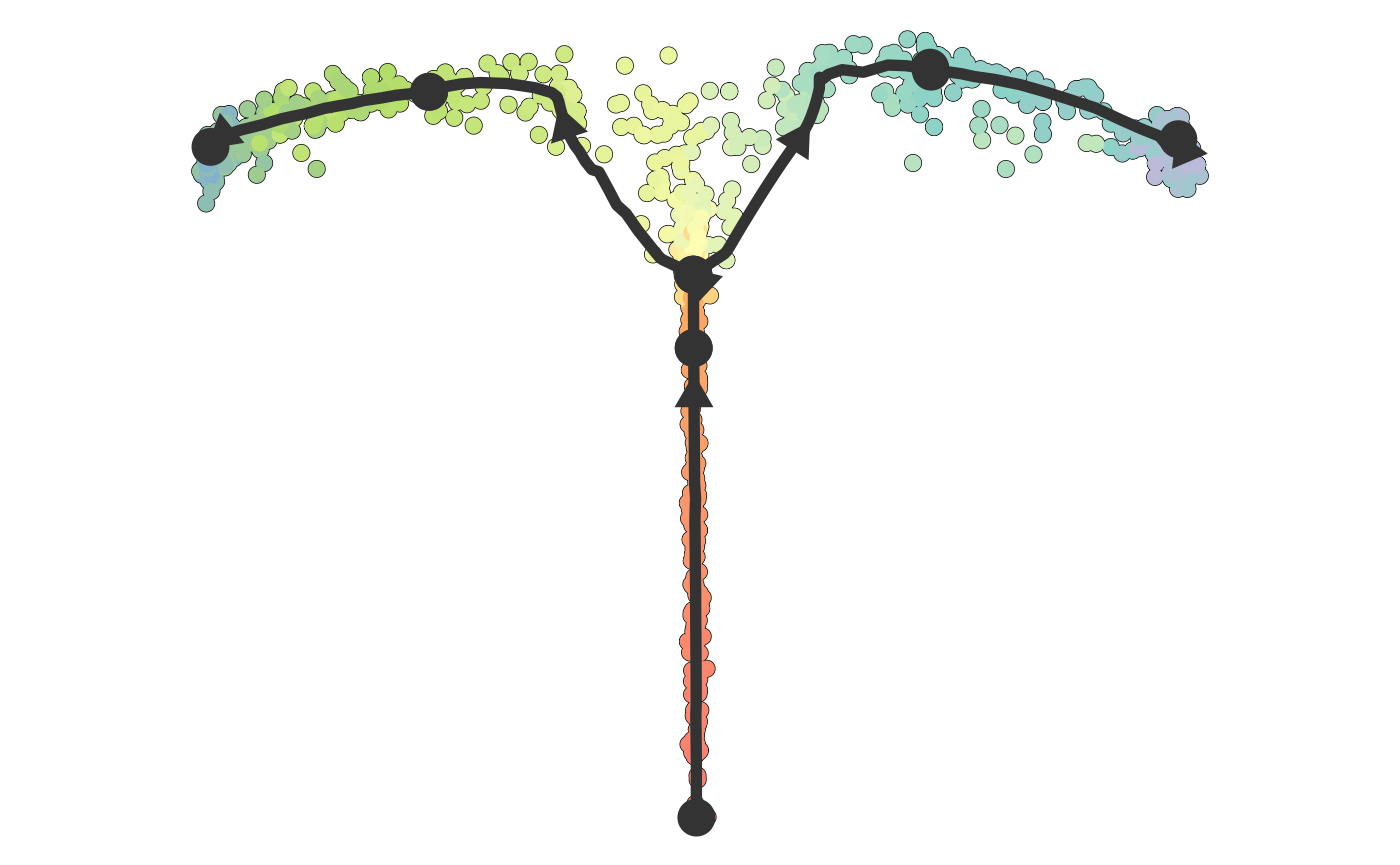plot_graph(dataset)
## Coloring by milestone
## Using milestone_percentages from trajectory